# Class 11 Maths NCERT Solutions for Chapter 6 Linear Inequalities Miscellaneous Exercise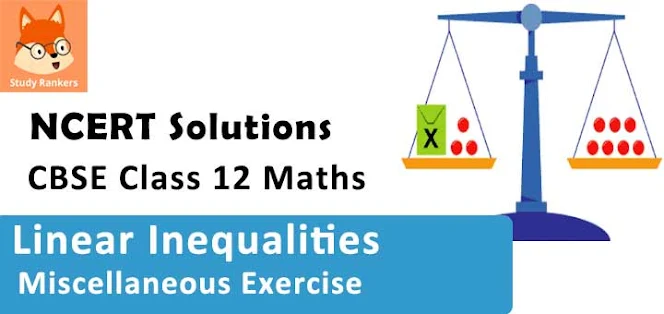### Linear Inequalities Miscellaneous Exercise Solutions

1. Solve the inequality 2 ≤ 3x – 4 ≤ 5

Solution

2 ≤ 3x – 4 ≤ 5
⇒ 2 + 4 ≤ 3x – 4 + 4 ≤ 5 + 4
⇒ 6 ≤ 3x ≤ 9
⇒ 2 ≤ ≤ 3
Thus, all the real numbers, x, which are greater than or equal to 2 but less than or equal to 3, are the solutions of the given inequality. The solution set for the given inequality is [2, 3].

2. Solve the inequality 6 ≤ –3(2x – 4) < 12

Solution

6 ≤ – 3(2x – 4) < 12
⇒ 2 ≤ –(2x – 4) < 4
⇒ –2 ≥ 2x – 4 > –4
⇒ 4 – 2 ≥ 2> 4 – 4
⇒ 2 ≥ 2x > 0
⇒1 ≥ > 0
Thus, the solution set for the given inequality is (0, 1].

3. Solve the inequality -3 ≤ 4 - 7x/2 ≤ 18

Solution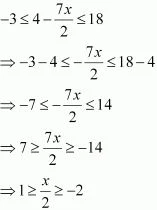⇒ 2  ≥ x  ≥ -4
Thus, the solution set for the given inequality is (-4, 2].

4. Solve the inequality -15 < 3(x-2)/5 ≤ 0
Solution
-15 < 3(x-2)/5 ≤ 0
⇒ –75 < 3(x – 2) ≤ 0
⇒ –25 < x – 2 ≤ 0
⇒ – 25 + 2 < ≤ 2
⇒ –23 < x ≤ 2
Thus, the solution set for the given inequality is (–23, 2].

5. Solve the inequality -12 < 4 - 3x/-5 ≤ 2
Solution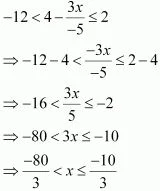Thus, the solution set for the given inequality is [-80/3, -10/3].

6. Solve the inequality 7 ≤ (3x + 11)/2 ≤ 11
Solution
7 ≤(3x + 11)/2 ≤ 11
⇒ 14 ≤ 3x + 11 ≤ 22
⇒ 14 - 11 ≤ 3x ≤ 22 - 11
⇒ 3 ≤ 3x ≤ 11
⇒1 ≤ x ≤ 11/3
Thus, the solution set for the given inequality is [1, 11/3] .

7. Solve the inequalities and represent the solution graphically on number line: 5x + 1 > –24, 5x – 1 < 24
Solution

5x + 1 > –24
⇒ 5> –25
⇒ > –5 …(1)
5x – 1 < 24
⇒ 5< 25
⇒ x < 5 …(2)
From (1) and (2), it can be concluded that the solution set for the given system of inequalities is (–5, 5). The solution of the given system of inequalities can be represented on number line as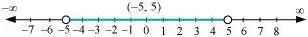8. Solve the inequalities and represent the solution graphically on number line: 2(x – 1) < x + 5, 3(x + 2) > 2 – x
Solution

2(x – 1) < x + 5
⇒ 2x – 2 < x + 5
⇒ 2x – x < 5 + 2
⇒ x < 7 …(1)
3(x + 2) > 2 – x
⇒ 3x + 6 > 2 – x
⇒ 3x + > 2 – 6
⇒ 4x > – 4
⇒ x > – 1 …(2)
From (1) and (2), it can be concluded that the solution set for the given system of inequalities is (–1, 7). The solution of the given system of inequalities can be represented on number line as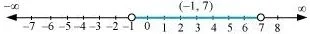9. Solve the following inequalities and represent the solution graphically on number line:
3x – 7 > 2(x – 6), 6 – x > 11 – 2x
Solution

3x – 7 > 2(x – 6)
⇒ 3x – 7 > 2x – 12
⇒ 3x – 2> – 12 + 7
⇒ x > –5 …(1)
6 – x > 11 – 2x
⇒ –x + 2x > 11 – 6
⇒ x > 5 …(2)
From (1) and (2), it can be concluded that the solution set for the given system of inequalities is (5, ∞). The solution of the given system of inequalities can be represented on number line as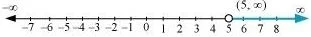10. Solve the inequalities and represent the solution graphically on number line: 5(2x – 7) – 3(2x + 3) ≤ 0, 2x + 19 ≤ 6x + 47

Solution

5(2x – 7) – 3(2x + 3) ≤ 0
⇒ 10x – 35 – 6x – 9 ≤ 0
⇒ 4x – 44 ≤ 0
⇒ 4x ≤ 44
⇒ x ≤ 11 …(1)
2x + 19 ≤ 6x + 47
⇒ 19 – 47 ≤ 6x – 2x
⇒ –28 ≤ 4x
⇒ –7 ≤ x …(2)
From (1) and (2), it can be concluded that the solution set for the given system of inequalities is [–7, 11]. The solution of the given system of inequalities can be represented on number line as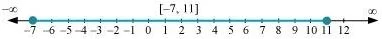11. A solution is to be kept between 68°F and 77°F. What is the range in temperature in degree Celsius (C) if the Celsius/Fahrenheit (F) conversion formula is given by F = (9/8)C + 32 ?

Solution

Since the solution is to be kept between 68°F and 77°F,
68 < F < 77
Putting F = (9/8)C + 32, we obtain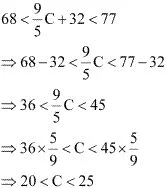Thus, the required range of temperature in degree Celsius is between 20°C and 25°C.

12. A solution of 8% boric acid is to be diluted by adding a 2% boric acid solution to it. The resulting mixture is to be more than 4% but less than 6% boric acid. If we have 640 litres of the 8% solution, how many litres of the 2% solution will have to be added?

Solution

Let x litres of 2% boric acid solution is required to be added.
Then, total mixture = (x + 640) litres
This resulting mixture is to be more than 4% but less than 6% boric acid.
∴2%x + 8% of 640 > 4% of (x + 640)
And, 2% + 8% of 640 < 6% of (x + 640)
2%x + 8% of 640 > 4% of (x + 640)
(2/100)x + (8/100) (640) > (4/100) (x + 640)
⇒ 2x + 5120 > 4x + 2560
⇒ 5120 – 2560 > 4x – 2x
⇒ 5120 – 2560 > 2x
⇒ 2560 > 2x
⇒ 1280 > x
2% + 8% of 640 < 6% of (x + 640)
(2/100)x + (8/100) (640) > (6/100) (x + 640)
⇒ 2x + 5120 < 6x + 3840
⇒ 5120 – 3840 < 6x – 2x
⇒ 1280 < 4x
⇒ 320 < x
∴320 < x < 1280
Thus, the number of litres of 2% of boric acid solution that is to be added will have to be more than 320 litres but less than 1280 litres.

13. How many litres of water will have to be added to 1125 litres of the 45% solution of acid so that the resulting mixture will contain more than 25% but less than 30% acid content?

Solution

Let x litres of water is required to be added.
Then, total mixture = (x + 1125) litres
It is evident that the amount of acid contained in the resulting mixture is 45% of 1125 litres.
This resulting mixture will contain more than 25% but less than 30% acid content.
∴30% of (1125 + x) > 45% of 1125
And, 25% of (1125 + x) < 45% of 1125
30% of (1125 + x) > 45% of 1125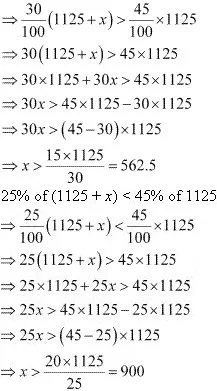∴ 562.5 < x < 900
Thus, the required number of litres of water that is to be added will have to be more than 562.5 but less than  900.

14. IQ of a person is given by the formula
IQ = (MA/CA) × 100
Where MA is mental age and CA is chronological age. If 80 ≤ IQ ≤ 140 for a group of 12 years old children, find the range of their mental age.

Solution

It is given that for a group of 12 years old children, 80 ≤ IQ ≤ 140  ...(i)
For a group of 12 years old children, CA = 12 years

IQ = (MA/12) × 100
Putting this value of IQ in (i) , we obtain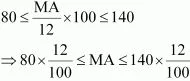⇒ 9.6 ≤ MA ≤ 16.8 ______
Thus, the range of mental age of the group of 12 years old children is 9.6 ≤ MA ≤ 16.8.